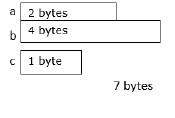# What is malloc in C language?

The C library memory allocation function void *malloc(size_t size) allocates the requested memory and returns a pointer to it.

## Memory allocation Functions

Memory can be allocated in two ways as explained below −Once memory is allocated at compile time, it cannot be changed during execution. There will be a problem of either insufficiency or else wastage of memory.

The solution is to create memory dynamically i.e. as per the requirement of the user during execution of program.

The standard library functions which are used for dynamic memory management are as follows −

• malloc ( )
• calloc ( )
• realloc ( )
• free ( )

## The Malloc() Function

This function is used for allocating a block of memory in bytes at runtime. It returns a void pointer, which points to the base address of allocated memory.

The syntax for malloc() is as follows −

void *malloc (size in bytes)

## Example 1

The following example shows the usage of malloc() function.

int *ptr;
ptr = (int * ) malloc (1000);
int *ptr;
ptr = (int * ) malloc (n * sizeof (int));

Note − It returns NULL, if memory is not FREE.

## Example program

Given below is the C program to demonstrate dynamic memory allocation function - malloc().

Live Demo

#include<stdio.h>
#include<stdlib.h>
void main(){
//Declaring variables and pointer//
int numofele,i;
int *p;
printf("Enter the number of elements in the array: ");
scanf("%d",&numofele);
//Declaring malloc function//
p = (int *)malloc(numofele * (sizeof(int)));
//Reading elements into array of pointers//
for(i=0;i<numofele;i++){
p[i]=i+1;
printf("Element %d of array is : %d",i,p[i]);
}
}

## Output

When the above program is executed, it produces the following result −

Enter the number of elements in the array: 4
Element 0 of array is : 1
Element 1 of array is : 2
Element 2 of array is : 3
Element 3 of array is : 4

## Example 2

Following is the C program to display the elements using dynamic memory allocation functions −

First five blocks should be empty, second five blocks should have the logic.

Live Demo

#include<stdio.h>
#include<stdlib.h>
void main(){
//Declaring variables and pointers,sum//
int numofe,i,sum=0;
int *p;
//Reading number of elements from user//
printf("Enter the number of elements : ");
scanf("%d",&numofe);
//Calling malloc() function//
p=(int *)malloc(numofe*sizeof(int));
/*Printing O/p -
We have to use if statement because we have to check if memory
has been successfully allocated/reserved or not*/
if (p==NULL){
printf("Memory not available");
exit(0);
}
//Printing elements//
printf("Enter the elements : ");
for(i=0;i<numofe;i++){
scanf("%d",p+i);
sum=sum+*(p+i);
}
printf("The sum of elements is %d",sum);
free(p);//Erase first 2 memory locations//
printf("Displaying the cleared out memory location : ");
for(i=0;i<numofe;i++){
printf("%d",p[i]);//Garbage values will be displayed//
}
}

## Output

When the above program is executed, it produces the following result −

Enter the number of elements : 5
Enter the elements :
12
10
24
45
67
The sum of elements is 158
Displaying the cleared out memory location :
7804032
0
7799120
0
67